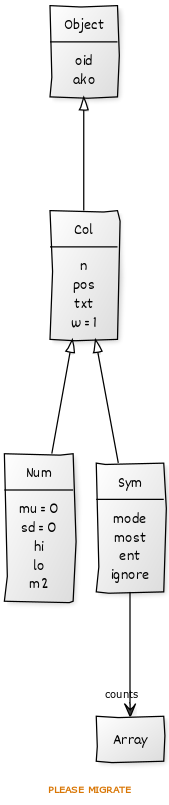# GOLD Methods

All GOLD methods receive a first argument holding the object. By convention, that variable is named `i` (but you could call it `self`, or `this`, if you prefer).

Recall that GOLD methods are functions with two upper case letters. Each method has to start with a class name. Also, GOLD constructors are functions with the same name as the class.

Constructor methods call the constructor of the super class methods. Each recursive call adds more attributes into an objects. For example, a call to `Sym` creates an object with `oid,isa,n,pos,txt,w,mode,most,ent,ignore,counts`.``````# this function is a
# built-in for GOLD
function new(i) { split("",i,"") }

# this constuctor is a
# built-in for GOLD
function Object(i)   {
new(i)
i["isa"] = "Object"
i["oid"] = ++OID
}

function Col(i,pos,txt,w) {
# this isa resets
# i.isa to "Col"
isa(Object(i))
i.n   = 0
i.txt = txt
i.w   = w
i.pos = pos="" ? 1 : pos
}

function Num(i,pos,txt,w) {
# this isa resets
# i.isa to "Num"
isa(Col(i,pos,txt,w))
i.hi  = -1e32
i.lo  =  1e32
i.mu = i.m2 = i.sd = 0
}

function Sym(i,pos,txt,w) {
# this isa resets
# i.isa to "Sym"
isa(Col(i,pos,txt,w))
i.mode = ""
i.most = 0
i.ent =""
i.ignore="?"
has(i,"counts")
}
``````

Note two other aspects of the above code.

• Firsly, arguments can be passed to super class constructors:
• For example, note how a call to `Sym(i,pos,txt,w)` passes those arguments to `Col(i,pos,txt,w).
• Secondly, all the calls to super constructors are wrapped in `isa`.
• This function calls the parent constructor then, after it returns, sets `i.isa` to the name of the current class constructor.

## Polymorphism

In GOLD, any call to `@Fun` (where the first letter of `Fun` is uppercase) is treated as polymorphic method call. For the call `@Fun(i,j,k,...)`:

• GOLD looks at the `isa` field of the first argument (which, in this case, is “`i`”);
• If `i.isa="Sym"` (say), then this call becomes `SayFun(i,j,k...)`.

For example, here are two definitions of `Add` (and note that `Add` is different to `Num` and `Sym`):

``````function NumAdd(i,x,          delta) {
x    += 0 # ensure "x" is a number
i.n  += 1
i.lo  = x < i.lo ? x : i.lo
i.hi  = x > i.hi ? x : i.hi
delta = x - i.mu
i.mu += delta/i.n
i.m2 += delta*(x - i.mu)
i.sd = (i.m2/(i.n-0.99999999))^0.5
return x
}

if( x == i.ignore ) return x
i.n++
tmp = i.counts[x] = i.counts[x] + 1
if(tmp > i.mode) {
i.most = tmp
i.mode = x
}
return  x
}
``````

The following code includes a polymorphic indirect function call (see `@Add`). Note that the `_thing` function is called twice: once for `Num` and once for `Sym` which leads to the generation of different objects (see the called to `@thing(t)`). The `@Add` call executes different methods (`SymAdd` or `NumAdd`), depending on what kind of object is being processed.

``````BEGIN {
_thing("Num")
_thing("Sym")
}
function _thing(thing, t,lst,s,j,k) {
s=" 9 2 5 4 12 7 8 11 9 3" \
" 7 4 12 5 4 10 9 6 9 4"
split(s, lst, " ")
@thing(t)
for(j=1; j<=10^4;j++) {
for(k in lst)
}
print(t.n)
}
``````

## Method Inheritance

Recall that constructor methods wrap the call to super class constructors in the `isa` function. As a side effect of the call, GOLD maintains a table

``````ISA[kid]=parent
``````

For example, if we used the above constructors to build a `Sym` instance then GOLD would know:

``````ISA["Sym"] = "Col"
ISA["Col"] = "Object"
``````

Using that information, GOLD’s polymorphic method lookup procedure implements method inheritance. In the following code:

• We are looking for a method “`f`” inside some object “`i`”, where `k=i.isa`.
• If we can not find a function “`k f`” inside the list of known functions (which GAWK stores in `FUNCTAB`)
• Then we jump up to the super class of “`i`”.
``````function my(i,f,    k,m) {
k = i["isa"]
while(k) {
m = k f
if (m in FUNCTAB) {
MY = m  # MY is the result
return ""
}
k = ISA[k]
}
print "E> failed method lookup on ["f"]";
exit 1
}
``````

GOLD using `my` as follows. Polymorphic methods calls in `*.gold` files like this:

``````function _some(f,    s,j) {
Some(s,32)
for(j=1;j<256;j++)
are converted into the following (in an associated `*.awk` file). Note that `@Add` (in the above) is converted to `@MY` (where `@MY` is set by the call `(my(s,"Add"))`.
``````function _some(f,    s,j) {